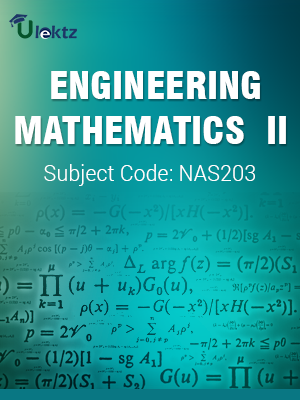uLektz apps

•My WalletMy Order
•My Profile
•My Connections
•My Books
•My Videos
•My Tests
•My Calender
•My Messages
•My Shopping Cart
•My Orders
•Account Settings
•Help

uLektz apps

# Book Details# Engineering Mathematics - II

Course Code:NAS203

Author:uLektz

Regulation:2013

Categories:Engineering Mathematics

Format :ePUB3 (DRM Protected)

Type :eBook

FREE

Description :Engineering Mathematics - II of NAS203 covers the latest syllabus prescribed by Dr. A.P.J. Abdul Kalam Technical University, Uttar Pradesh for regulation 2013. Author: uLektz, Published by uLektz Learning Solutions Private Limited.

Note : No printed book. Only ebook. Access eBook using uLektz apps for Android, iOS and Windows Desktop PC.

##### Topics
###### Unit - 1: Differential Equations

1.1 Linear differential equations of nth order with constant coefficients,

1.2 Complementary function and Particular integral, Simultaneous linear differential equations,

1.3 Solution of second order differential equations by changing dependent & independent variables, Normal form,

1.4 Method of variation of parameters, Applications to engineering problems (without derivation).

###### Unit - 2: Series Solution and Special Functions

2.1 Series solution of second order ordinary differential equations with variable coefficient (Frobenius method)

2.2 Bessel and Legendre equations and their series solutions

2.3 Properties of Bessel function and Legendre polynomials

###### Unit - 3: Laplace Transform

3.1 Laplace transform, Existence theorem, Laplace transforms of derivatives and integrals, Initial and final value theorems

3.2 Unit step function, Dirac- delta function

3.3 Laplace transform of periodic function

3.4 Inverse Laplace transform, Convolution theorem

3.5 Application to solve simple linear and simultaneous differential equations

###### Unit - 4: Fourier Series and Partial Differential Equations

4.1 Periodic functions, Fourier series of period 2Π,

4.2 Euler’s Formulae, Functions having arbitrary periods,

4.3 Change of interval, Even and odd functions,

4.4 Half range sine and cosine series,

4.5 Harmonic analysis.

4.6 Solution of first order partial differential equations by Lagrange’s method,

4.7 Solution of second order linear partial differential equations with constant coefficients.

###### Unit - 5: Applications of Partial Differential Equations

5.1 Classification of second order partial differential equations,

5.2 Method of separation of variables for solving partial differential equations,

5.3 Solution of one and two dimensional wave and heat conduction equations,

5.4 Laplace equation in two dimension,

5.5 Equation of transmission lines.

### Related Books